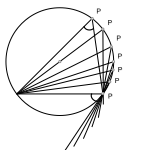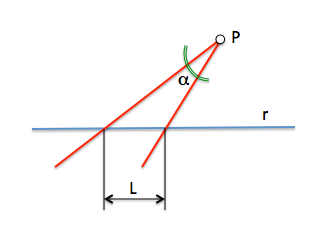# Metric geometry : Arco able on a segment

The relationship between the inscribed angle and the central angle in a circle allows for a locus of great importance for numerous applications in metric geometry; This locus is referred arc able.Circumference points that are vertices of triangles whose common basis is a chord of the circumference have the property to be associated at the same angle vertex, which corresponds to half of the central angle covered by said base.

This property allows you to state the definition of the locus called Arco able on a segment.

Arc able to segment AB seen under an angle α given is the locus of points in the plane from which the segment AB is the same angle α.

## Arch construction capable

The point P observes the segment AB (rope circumference) at a certain angle (alfa). Hovering over this circumference the angle remains invariant.

PA and PB segments vary in length so, but the angle. This concept allows determining an elementary construction, given segment AB and the angle alpha, determine the center of the circle described.

If the point P is moved to coincide with the point B, AP segment becomes AB, BP and the segment becomes tangent to the circumference, so the tangent in B form alpha degrees with the segment AB.

The tangent and the radius passing through the contact point are orthogonal

To construct the arc capable, or determine the circumference, simply determine its center as the intersection of the perpendicular to the line perpendicular to the tangent at B (to determine beforehand)

The arc capable of 90 degrees is a semicircle.

## Arch applications capable

Besides being used to solve problems loci, is especially useful as a tool for proving theorems classical metric geometry.

### Application to geometric constructions

The arc capable of greater interest is 90 grados, namely, the right angle. This locus is of great use in solving basic problems of tangents and subsequently used in harmonic relationships.
As the tangent and the radius passing through the contact point are orthogonal, We can use a bow capable of 90 determining degrees from a tangent to a circle. Simply determine an arc able (semicircumference) between the point from which we draw the tangent and the center C of the circle to which the line must be tangent. T intersection point is the point of tangency sought.

### Application in demonstrations

Theorems shows the angles are shown in the arc capable of 90 grades have immediate application. For example, a classical theorem are:

The orthocenter of a triangle is the incenter of the triangle orthic.

The orthocenter is the point of intersection of the altitudes of the triangle ABC, lines through a vertex and the foot of the perpendicular to the opposite side (H). This point is therefore in the intersection of two arcs capable.

The triangle orthic is passing through the foot of the heights, incentro and the point of intersection of the bisectors.

From the figure we can deduce the above theorem, simply showing that marked angles are equal to be able arches on the same segment in different circles shown.

## Training

1-.Determine a point P within the given triangle, from which three sides look the same angle. (Problem)

2-.Given a point P and a line r, situated at a distance of 38mm, draw a 45 degree angle with vertex P r intercept in a segment of 30mm. In place of the generic form straight lines that pass through P at an angle alpha, which intersects the line R as a segment of length L. (Problem)3.- Construct a triangle known side , its opposite angle and a third condition.

Data (Side c, to, Ángulo A).

Unknown (Construir Triángulo ABC)

4.- Building a known triangle the hypotenuse and a second condition
Data (A Hypotenuse, ángulo C).
Unknown (Construct triangle ABC)